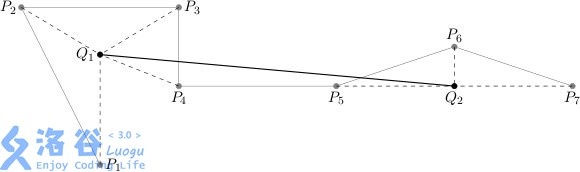# P3517 [POI2011]WYK-Plot

• 0通过
• 28提交
• 题目提供者 洛谷
• 评测方式 云端评测
• 标签 二分答案 倍增 POI 2011 Special Judge
• 难度 尚无评定
• 时空限制 10000ms / 512MB
• 提示：收藏到任务计划后，可在首页查看。

## 题目描述

We call any sequence of points in the plane a plot.

We intend to replace a given plot $(P_1,\cdots,P_n)$ with another that will have at most $m$ points ($m\le n$) in such a way that it "resembles" the original plot best.

The new plot is created as follows. The sequence of points $P_1,\cdots,P_n$ can be partitioned into $s$ ($s\le m$) contiguous subsequences:

$(P_{k_0+1},\cdots,P_{k_1}),(P_{k_1+1},\cdots,P_{k_2}),\cdots,(P_{k_{s-1}+1},\cdots,P_{k_s})$ where $0=k_0<k_1<k_2<\cdots<k_s=n$,and afterwards each subsequence $(P_{k_{i-1}+1},\cdots,P_{k_i})$, for $i=1,\cdots,s$,is replaced by a new point $Q_i$.

In that case we say that each of the points $P_{k_{i-1}+1},\cdots,P_{k_i}$ has been contracted to the point $Q_i$.

As a result a new plot, represented by the points $Q_1,\cdots,Q_s$, is created.

The measure of such plot's resemblance to the original is the maximum distance of all the points $P_1,\cdots,P_n$ to the point it has been contracted to:

$max_{i=1,\cdots,s}(max_{j=k_{i-1}+1,\cdots,k_i}(d(P_j,Q_i)))$ where $d(P_j,Q_i)$ denotes the distance between $P_j$ and $Q_i$, given by the well-known formula:

$d((x_1,y_1),(x_2,y_2))=\sqrt{(x_2-x_1)^2+(y_2-y_1)^2}$An exemplary plot $P_1,\cdots,P_7$ and the new plot $(Q_1,Q_2)$, where $(P_1,\cdots,P_4)$ are contracted to $Q_1$, whereas $(P_5,P_6,P_7)$ to $Q_2$.

For a given plot consisting of $n$ points, you are to find the plot that resembles it most while having at most $m$ points, where the partitioning into contiguous subsequences is arbitrary.

Due to limited precision of floating point operations, a result is deemed correct if its resemblance to the given plot is larger than the optimum resemblance by at most $0.000001$.

给定n个点，要求把n个点分成不多于m段，使得求出每段的最小覆盖圆的半径后，最大的半径最小。

## 输入输出格式

输入格式：

In the first line of the standard input there are two integers $n$ and $m$, $1\le m\le n\le 100\ 000$, separated by a single space.

Each of the following $n$ lines holds two integers, separated by a single space.

The $(i+1)$-th line gives $x_i$,$y_i$,$-1\ 000\ 000\le x_i,y_i\le 1\ 000\ 000$ denoting the coordinates $(x_i,y_i)$ of the point $P_i$.

输出格式：

In the first line of the standard output one real number $d$ should be printed out, the resemblance measure of the plot found to the original one.

In the second line of the standard output there should be another integer $s$, $1\le s\le m$.

Next, the following $s$ lines should specify the coordinates of the points $Q_1,\cdots,Q_s$,one point per line.

Thus the $(i+2)$-th line should give two real numbers $u_i$ and $v_i$, separated by a single space, that denote the coordinates $(u_i,v_i)$ of the point $Q_i$.All the real numbers should be printed with at most 15 digits after the decimal point.

## 输入输出样例

输入样例#1： 复制
7 2
2 0
0 4
4 4
4 2
8 2
11 3
14 2
输出样例#1： 复制
3.00000000
2
2.00000000 1.76393202
11.00000000 1.99998199

## 说明

给定n个点，要求把n个点分成不多于m段，使得求出每段的最小覆盖圆的半径后，最大的半径最小。

提示
标程仅供做题后或实在无思路时参考。
请自觉、自律地使用该功能并请对自己的学习负责。
如果发现恶意抄袭标程，将按照I类违反进行处理。